# Geometric Properties of Objects: Help and Review Chapter Exam

Exam Instructions:

Choose your answers to the questions and click 'Next' to see the next set of questions. You can skip questions if you would like and come back to them later with the yellow "Go To First Skipped Question" button. When you have completed the practice exam, a green submit button will appear. Click it to see your results. Good luck!

### Page 1

#### Question 2 2. What is the area of this trapezoid?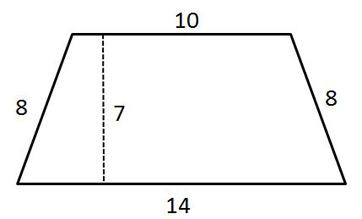#### Question 3 3. In the figure below, Angle 7 = 15x and Angle 10 = 9x + 30. Determine the measure of Angle 10.#### Question 4 4. The pictured shape is a: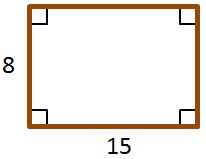#### Question 5 5. Given the figure below, Angle 3 = 140y and Angle 5 = 80y + 70. Determine the value of y.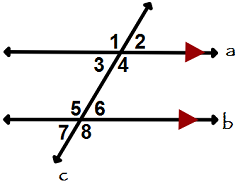### Page 2

#### Question 6 6. Which two lines are parallel?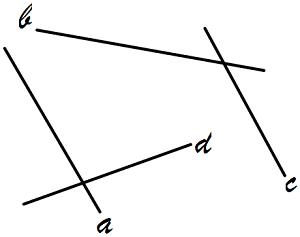#### Question 8 8. In the similar rectangles pictured below, what is y?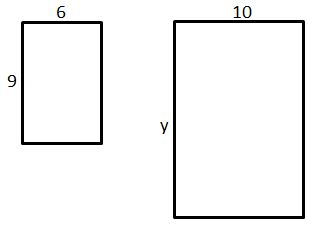#### Question 9 9. Which of the following rectangles represents a scale factor of 3 from the rectangle pictured below?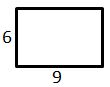### Page 3

#### Question 11 11. What is the relationship between Angle 2 and Angle 7?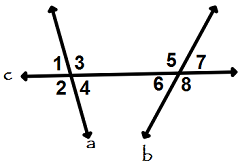#### Question 12 12. If the two triangles pictured below are similar, then what is x?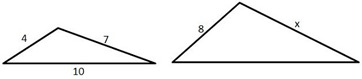#### Question 15 15. What is the perimeter of the pictured square?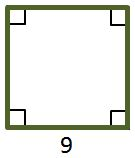### Page 4

#### Question 16 16. What is the relationship between Angle 1 and Angle 7?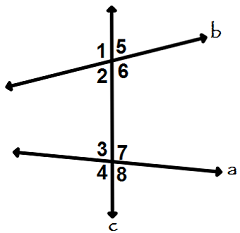#### Question 17 17. Josh claims that these triangles are similar by SSS. Do you agree or disagree with Josh?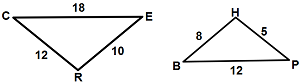### Page 5

#### Question 21 21. Mary claims that the triangles below are similar by AA. Do you agree or disagree with Mary?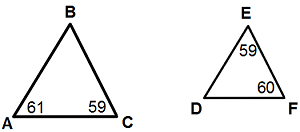#### Question 22 22. In the figure below, Angle 13 = 10y and Angle 11 = 3y + 11. Determine the measure of Angle 16.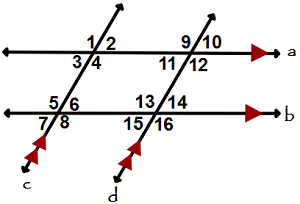#### Question 23 23. The pictured shape is all of the following EXCEPT a: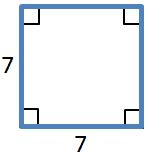#### Question 25 25. If triangle MNO is congruent to triangle PQR, then what is the measure of angle x?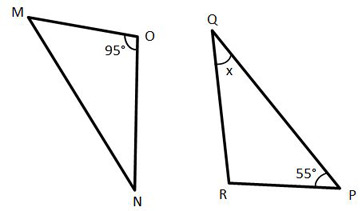### Page 6

#### Question 27 27. Determine if triangle VEZ is similar to triangle MAG.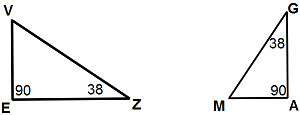#### Question 28 28. Which line is the transversal?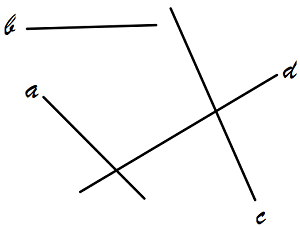#### Question 29 29. Study the given diagram. Find the value of x, the distance between T and E.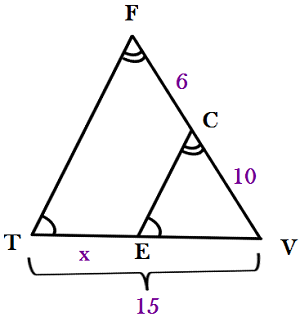#### Question 30 30. What is the area of this parallelogram?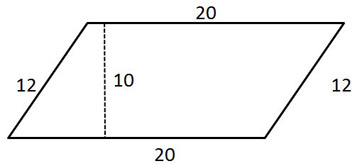#### Geometric Properties of Objects: Help and Review Chapter Exam Instructions

Choose your answers to the questions and click 'Next' to see the next set of questions. You can skip questions if you would like and come back to them later with the yellow "Go To First Skipped Question" button. When you have completed the practice exam, a green submit button will appear. Click it to see your results. Good luck!

Support